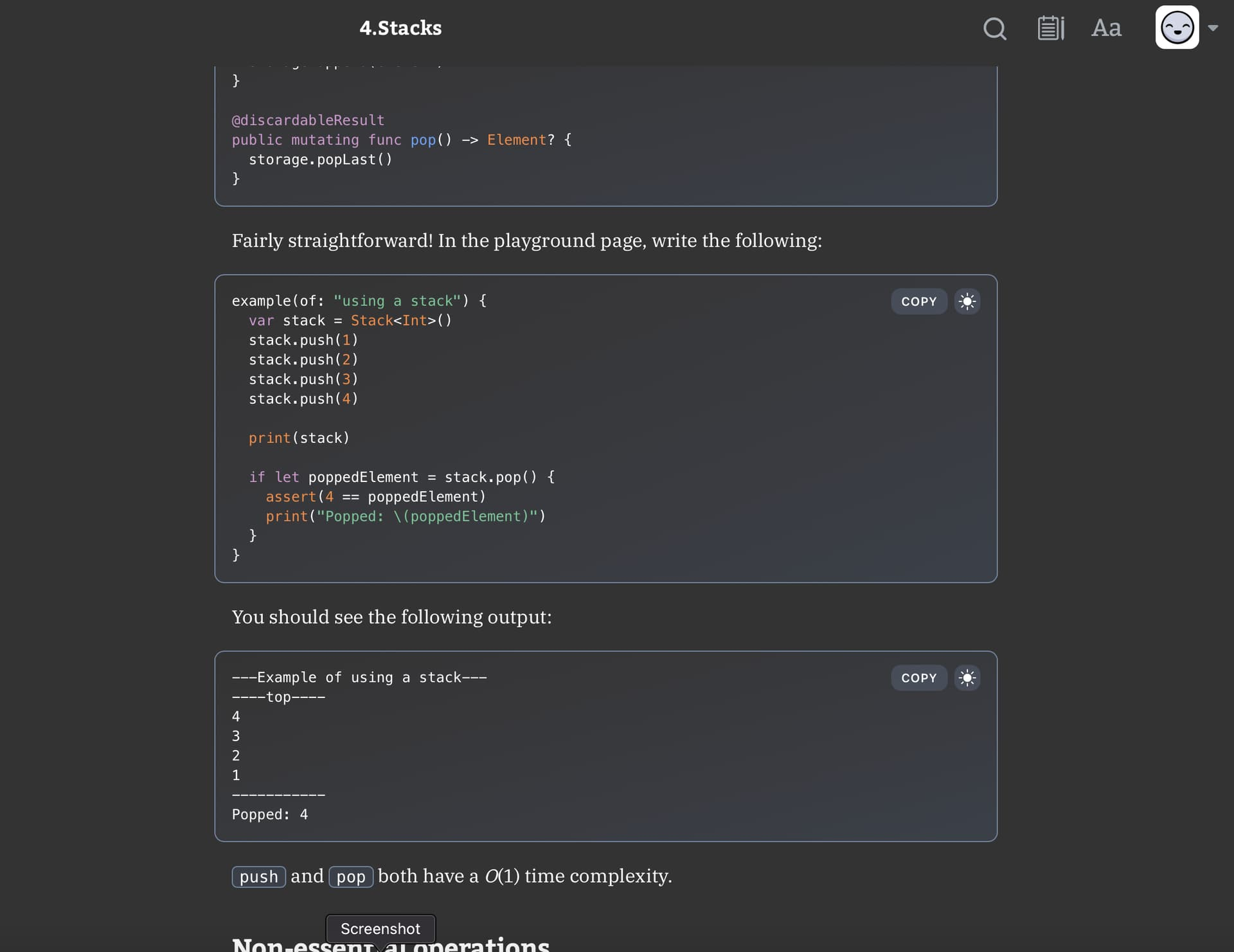# CH4, Stacks reviewing code

Give me a rundown on this please, check to make sure I did this correctly.

``````// Copyright (c) 2021 Razeware LLC

public struct Stack<Element> {

private var storage: [Element] = []

public init() { }

public mutating func push(_ element: Element) {
storage.append(element)
}

public mutating func pop() -> Element? {
storage.popLast()
}

public func peek() -> Element? {
storage.last
}

public var isEmpty: Bool {
peek() == nil
}

public init(_ elements: [Element]) {
storage = elements
}

}

extension Stack: CustomDebugStringConvertible {

public var debugDescription: String {
"""
----top----
\(storage.map { "\(\$0)" }.reversed().joined(separator: "\n"))
-----------
"""
}
}

example(of: "using a stack") {
var stack = Stack<Int>()
stack.push(1)
stack.push(2)
stack.push(3)
stack.push(4)

print(stack)

if let poppedElement = stack.pop() {
assert(4 == poppedElement)
print("Popped: \(poppedElement)")
}
}

example(of: "initializing a stack from an array") {
let array = ["A", "B", "C", "D"]
var stack = Stack(array)
print(stack)
stack.pop()
}

extension Stack: ExpressibleByArrayLiteral {
public init(arrayLiteral elements: Element...) {
storage = elements
}
}

example(of: "initializing a stack from an array literal") {
var stack: Stack = [1.0, 2.0, 3.0, 4.0]
print(stack)
stack.pop()
}

``````im having trouble with this section. Basically I can’t get it to run, always has a error. I have taken what was said, tried putting the steps in a different orders and still wasn’t able to get it working. Can you please help with this section.

Hey @lac_ekim, I tried copying the text verbatim to see if there were some typos - doesn’t seem to be any issues.

Could you try copying the text and pasting into the editor directly?

Hello Kelvin, having some issues, please review.

``````public struct Stack<Element> {

private var storage: [Element] = []

public init() { }
}

extension Stack: CustomDebugStringConvertible {

public var debugDescription: String {
"""
----top----
\(storage.map { "\(\$0)" }.reversed().joined(separator: "\n"))
-----------
"""
}

public mutating func push(_ element: Element) {
storage.append(element)
}

public mutating func pop() -> Element? {
storage.popLast()
}

public func peek() -> Element? {
storage.last
}

public var isEmpty: Bool {
peek() == nil
}

public init(_ elements: [Element]) {
storage = elements
}

}

example(of: "using a stack") {
var stack = Stack<Int>()
stack.push(1)
stack.push(2)
stack.push(3)
stack.push(4)

print(stack)

if let poppedElement = stack.pop() {
assert(4 == poppedElement)
print("Popped: \(poppedElement)")
}
}

example(of: "initializing a stack from an array") {
let array = ["A", "B", "C", "D"]
var stack = Stack(array)
print(stack)
stack.pop()
}

extension Stack: ExpressibleByArrayLiteral {
public init(arrayLiteral elements: Element...) {
storage = elements
}
}

example(of: "initializing a stack from an array literal") {
var stack: Stack = [1.0, 2.0, 3.0, 4.0]
print(stack)
stack.pop()
}
``````

That is my final thing by this lesson. However when I go look at the final code in the code I downloaded, alg-materials/04-Stacks/Projects/ I have two folders, one being starter and one final. This is what it has for final

``````example(of: "using a stack") {
var stack = Stack<Int>()
stack.push(1)
stack.push(2)
stack.push(3)
stack.push(4)

print(stack)

if let poppedValue = stack.pop() {
assert(4 == poppedValue)
print("Popped: \(poppedValue)")
}
}

example(of: "initializing a stack from an array literal") {
var stack: Stack = [1.0, 2.0, 3.0, 4.0]
print(stack)
stack.pop()
}

example(of: "initializing a stack from an array") {
let array = ["A", "B", "C", "D"]
var stack = Stack(array)
print(stack)
stack.pop()
}

``````

If you wouldn’t mind explaining what im seeing compared to the other?

``````extension Stack: ExpressibleByArrayLiteral {
public init(arrayLiteral elements: Element...) {
storage = elements
}
}

``````

I understand it but am wondering why I did it this way instead of this way:

``````public struct Stack<Element> {

private var storage: [Element] = []

public init() { }
}

extension Stack: CustomDebugStringConvertible {

public var debugDescription: String {
"""
----top----
\(storage.map { "\(\$0)" }.reversed().joined(separator: "\n"))
-----------
"""
}

public mutating func push(_ element: Element) {
storage.append(element)
}

public mutating func pop() -> Element? {
storage.popLast()
}

public func peek() -> Element? {
storage.last
}

public var isEmpty: Bool {
peek() == nil
}

public init(_ elements: [Element]) {
storage = elements
}

}
``````

They are all in the stack, however there in extensions. please go into that farther of why this is the way it is?